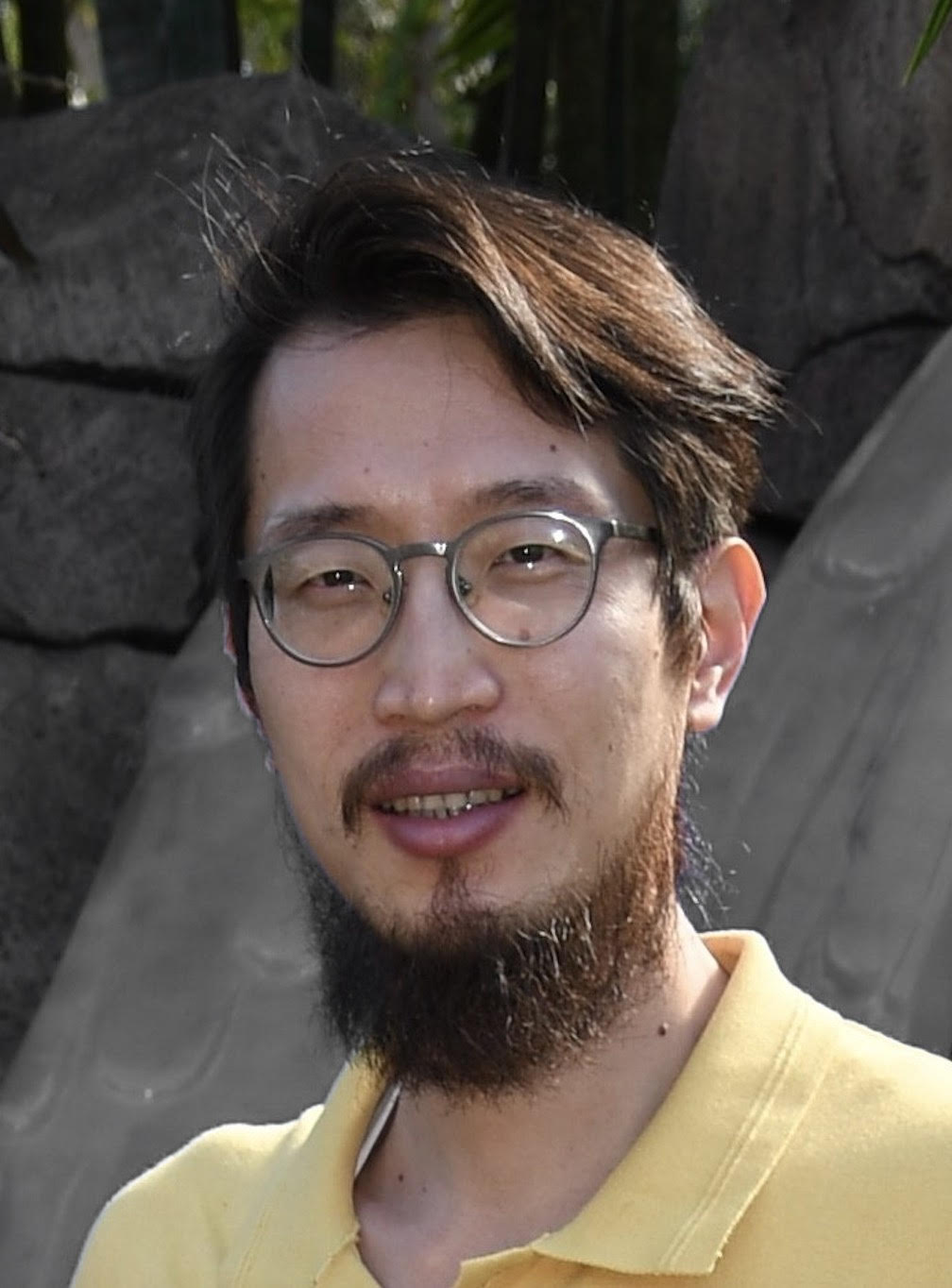##### Pronounce xiangxiong

• Selected recent work:
• S. Zheng, H. Yang,  and X. Zhang, On the convergence of orthogonalization-free conjugate gradient method for extreme eigenvalues of Hermitian matrices: a Riemannian optimization interpretation. PDF
• Z. Chen, J. Lu, Y. Lu and X. Zhang, On the convergence of Sobolev gradient flow for the Gross-Pitaevskii eigenvalue problem. arXiv
• X. Liu, J. Shen and X. Zhang, An efficient and robust SAV based algorithm  for discrete gradient systems arising from optimizations. PDF
• C. Liu, Y. Gao and X. Zhang, Structure preserving schemes for Fokker-Planck equations of irreversible processes.  arXiv
• S. Zheng, W. Huang, B. Vandereycken and X. Zhang, Riemannian optimization using three different metrics for Hermitian PSD fixed-rank constraints: an extended version. arXiv PDF
• C. Fan, X. Zhang and J. Qiu, Positivity-preserving high order finite difference WENO schemes for the compressible Navier-Stokes equations, Journal of Computational Physics, 467 (2022): 111446. PDF
• H. Li, D. Appelö and X. Zhang, Accuracy of spectral element method for  wave, parabolic and Schrödinger equations, SIAM Journal on Numerical Analysis 60(1):339–363, 2022. PDF. See Section 2.8 in here for detailed discussion of Neumann b.c..
• L. Cross and X. Zhang, On the monotonicity of high order discrete Laplacian, submitted. PDF